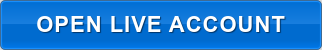A lot in Forex trading basically refers to the size of a trade or the amount that a trader trades at any given time.

Because the lot size directly influences the risk you're taking, it's the first thing you need to understand fully, before identifying your entry or exit points. Even the best trading strategy will fail you if you don’t have a clear idea of the lot size you should be using.

You will understand more as we go along.

## 4 Types of Lots DefinedA standard lot is equivalent to 100,000 units of the Base currency.

A mini lot is 10% of the standard lot or 10,000 units of the Base currency.

A micro lot is 1% of the standard lot of 1,000 units of the Base currency.

A nano lot is 0.1% of the standard lot of 1,000 units of the Base currency.

It is often the case now that the default is the standard lot. You can trade as small as a micro lot on Fullerton Markets’ MT4 platform.

Let's say you want to trade 1 lot or 100,000 units of AUD/USD, the size of the trade is equivalent to AUD 100,000.

## Understanding Pips and Value per Pip

A pip or percentage in point is the change in value between two currencies. For yen pairs, 1 pip is 0.01 while for most of the other currencies, 1 pip is 0.0001.

If the value of EUR/USD opens at 1.1385, for example, and closes at 1.1395, then the result is 10 pips difference. Another example is USD/JPY opens at 107.400, and closes at 107.420, the result is 2 pips difference.

In terms of calculating the value per pip, the formula is as follows:

Pip value in Counter/Quote currency = (Pip in decimal X 100,000)

For example:

USD/JPY, Value per pip per lot = (0.01x100,000) = JPY1,000.

The good news is that you don’t need to do this manually. You can simply use any of the pip value calculators available online.

Why should you care about lots, pip, and pip value? Because you need all three to calculate profit and loss.

Profit/Loss = Number of Pips x Value per Pip x Lot size

For example, when you buy EUR/USD at 1.32140 and close it at 1.32250:

Number of Pips = 11

Value per Pip = USD\$10

Lot size = 1 (standard lot)

Profit = 11 x USD\$10 x 1 = USD\$110

Your profit or loss will be USD\$110.

Another example, when you sell USD/JPY at 107.55 and close it at 107.95:

Number of Pips = 40

Value per Pip (JPY) = JPY\$1,000

Lot size = 1 (standard lot)

Profit = 40 x JPY1,000 x 1 = JPY40,000

Your Loss will be JPY40,000 and has to be converted to the base currency (USD) which is:
JPY40,000/107.95=USD370.54

Now, do you see why it's important to understand lot size in Forex?

## Use a Position Size Calculator

Rather than doing manual calculations, you can take a shortcut and use one of the many position size calculators online. This tool will tell you what position size to trade based on your risk level, your choice of currency pair, and stop-loss or pips. Here's one from BabyPips.

Ready to grow your wealth in the world's largest financial market? No better place to start than right here with us! Begin trading with Fullerton Markets today by opening an account:You might be interested in: How to Better Overcome [or Mitigate] Forex Trading Risks and Profit More